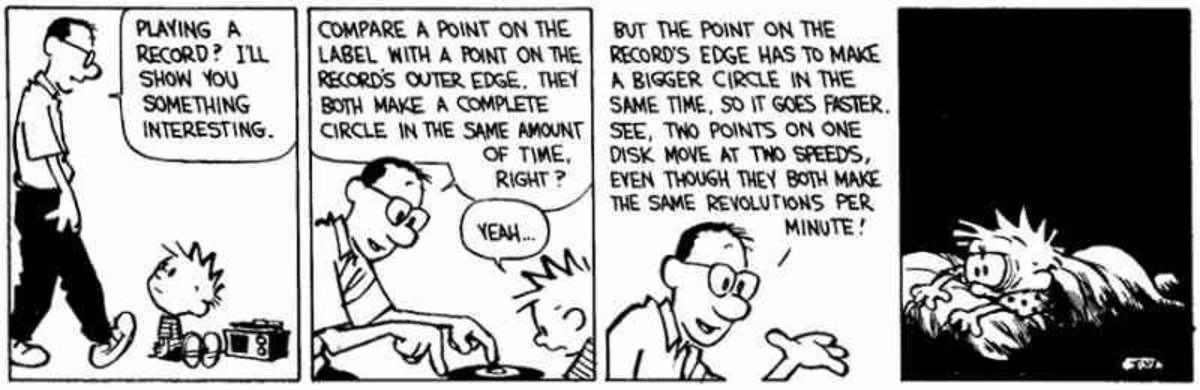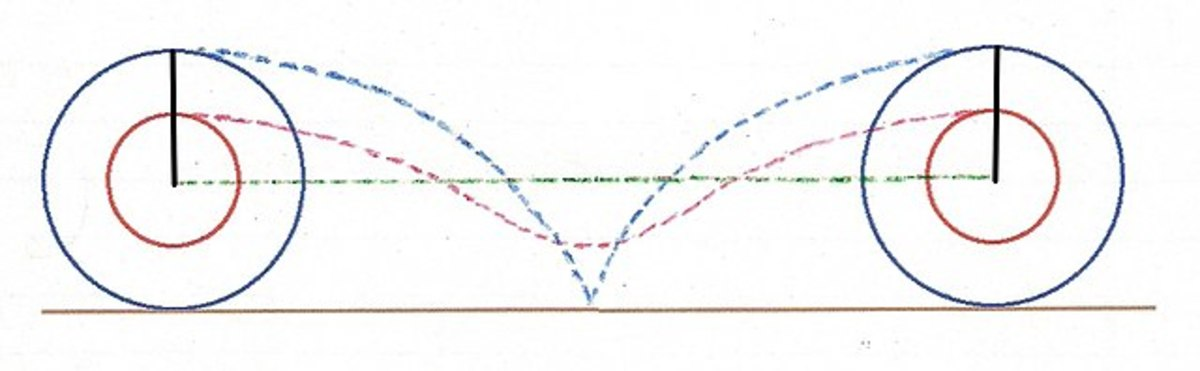# Interesting Paradoxes: Aristotle's Wheel

Mohammad Yasir is a physics graduate from the University of Delhi and currently enrolled in the master's programme at IIT.

## Explaining Aristotle's Wheel

Philosophy and science are often inseparable. The Aristotle's Wheel paradox is another testimonial to that fact. Indeed, being a Physics major, I find myself most fascinated by problems that carry a scientific weight behind them. But worry not! Making these articles fun and easy to understand is still my primary goal.

Aristotle's wheel is a very popular paradox. It is only the nomenclature which you might find singular. Let us take a glimpse at what the problem is saying.

Note: In the description given below, "greater" and "smaller" refer to circles formed by two points: one lying on the outer edge of a wheel, while the other one lying somewhere inside and thus, inscribing a smaller circle when the wheel is rotated.

Now since there is no stopping of the greater for the smaller so that it [the greater] remains for an interval of time at the same point, and since the smaller does not leap over any point, it is strange that the greater traverses a path equal to that of the smaller, and again that the smaller traverses a path equal to that of the larger.

— Author disputed. This problem was published in Mechanica, most likely authored by Aristotle

### What Is the Paradox of Aristotle's Wheel?

Here is what the paradox states. Suppose you take two points on a wheel, one on its extreme outer edge, and the other one somewhere in the middle. Let the wheel make one complete revolution without sliding on the ground. Then, as shown in the image above, both the points cover the same distance across the ground, which should, in theory, be equal to the circumferences of the circle they make as the wheel rotates. You might already be seeing the paradox here but if not, let me break it down.

The point on the edge of the wheel must make a bigger circle than the point that lies inside. And yet, they travel the same distance, which makes the distance travelled by the latter greater than the circumference of its corresponding circle. So, it must not cover the same distance as the outer one. Thus, somehow, the wheel is rotating at a constant speed and retaining its shape but the two points on it cover different distances through the course of its motion.

So, what's going on?

Scroll to ContinueThis paradox was also featured in a famous Calvin and Hobbes comic strip. Luckily, after this article, you won't be lying in the dark like Calvin.

## What's the Answer?

Multiple analyses and resolutions have emerged to this problem. On the Wikipedia page about Aristotle's Wheel Paradox, three solutions have been given. However, only one of those appealed to me. That is the one I am trying to explain in layman's terms here.

Recall that the shortest distance between two points is a straight line (turn your back, physicists, we are not talking about curved space here). And this is the path that the center of the wheel takes to reach its target. It simply moves in a straight line, something we physicists refer to as translatory motion. But points away from the circle do a little bit more than that. They perform rotational motion about the center as well.The two points cover different distances as the wheel rotates. Instead of a straight line, they go through a cycloid path.Wikimedia Commons

## What Does Rotation + Translation Get You?

Now how does knowing about rotation and translation help, you ask? It is simple. When a point is performing rotational motion along with translatory motion, its path can prove extraordinarily complex. It no longer travels in a straight line. In fact, if the situation here was just a little bit different, then the resulting motion of the points would have been so complicated that I would have been unable to explain it. Luckily, for a simple, two-dimensional case, it's quite simple to understand, especially given the image above.

Notice how as the wheel rotates, the path of the inner point, represented by the red dotted line, is in the form of a cycloid. The outer point does something similar, but follows a lengthier, curvier path. It even touches the ground once just as you might expect it to. Thus, even though the wheel retains its shape, the two points do move at differing velocities and follow different paths over the course of the wheel's rotation. The distances traveled by them correspond to these paths and are not equal as you might expect. That is how the paradox gets resolved.

## An Alternate Answer for Physics Enthusiasts

Another way of looking at this is in terms of linear and angular velocities. Those who have studied rotational motion in high school will easily understand this. The angular velocities of the two points are equal and thus, they cover the same angular distance of 3600 or 2π. On the other hand, since their linear velocities are different, they cover a different distance in terms of linear motion, given by the cycloid curves in the image above.

## The Resolution: There Is No Paradox

Thus, there is no paradox here since the two points travel different distances as the wheel rotates. Their angular distance is the same but in terms of linear motion, they go through a cycloid path which is smaller and more direct for a point lying closer to the center. The shorter the distance from the center, the more direct is the path taken.